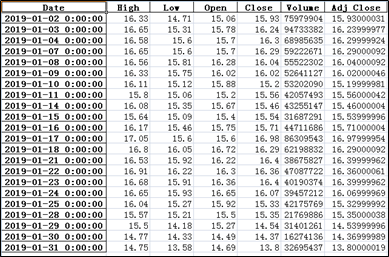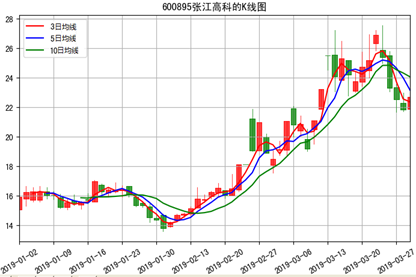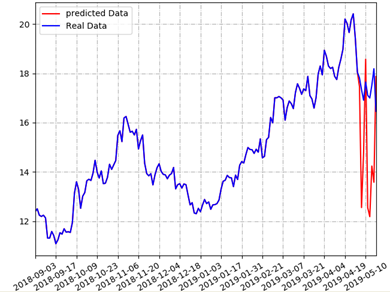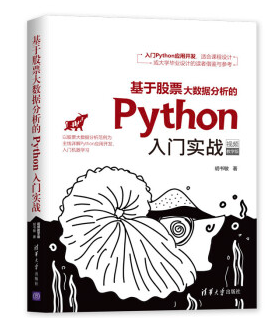# 用Python爬取股票数据，绘制K线和均线并用机器学习预测股价（来自我出的书） - hsm_computer - 博客园```1    # coding=utf-8
2    from pandas_datareader import data as pdr
3    import yfinance as yf
4    yf.pdr_override()
5    code='600895.ss'
6    stock = pdr.get_data_yahoo(code,'2019-01-02','2019-02-01')
7    print(stock)    # 输出内容
8    # 保存为excel和csv文件
9    stock.to_excel('D:\\stockData\\ch5\\'+code+'.xlsx')
10    stock.to_csv('D:\\stockData\ch5\\'+code+'.csv')``````1    # coding=utf-8
2    from pandas_datareader import data as pdr
3    import yfinance as yf
4    yf.pdr_override()
5    stockCodeList = []
6    stockCodeList.append('600007.ss')      # 沪股“中国国贸”
7    stockCodeList.append('000001.sz')     # 深股“平安银行”
8    stockCodeList.append('2318.hk')       # 港股“中国平安”
9    stockCodeList.append('IBM')         # 美股，IBM，直接输入股票代码不带后缀
10    for code in stockCodeList:
11        # 为了演示，只取一天(2019-01-02)的交易数据
13        print(stock)```

#### 2 用matplotlib绘制k线和均线

K线是由开盘价、收盘价、最高价和最低价这四个要素构成。在得到上述四个值之后，首先用开盘价和收盘价绘制成一个长方形实体。随后根据最高价和最低价，把它们垂直地同长方形实体连成一条直线，这条直线就叫影线。如果再细分一下，长方形实体上方的就叫上影线，下方的就叫下影线。通过K线可以形象地记录价格变动的情况，常用的有日K线，周K线和月K线。

```1    # !/usr/bin/env python
2    # coding=utf-8
3    from pandas_datareader import data as pdr
4    import pandas as pd
5    import matplotlib.pyplot as plt
6    from mpl_finance import candlestick2_ochl
7    from matplotlib.ticker import MultipleLocator
8    import yfinance as yf
9    yf.pdr_override()
10    # 根据指定代码和时间范围获取股票数据
11    code='600895.ss'
12    stock.drop(stock.index[len(stock)-1],inplace=True)
13    # 保存在本地
14    stock.to_csv('D:\\stockData\ch7\\600895.csv')
16    # 设置窗口大小
17    fig, ax = plt.subplots(figsize=(10, 8))
18    xmajorLocator   = MultipleLocator(5)     # 将x轴主刻度设置为5的倍数
19    ax.xaxis.set_major_locator(xmajorLocator)
20    # 调用方法绘制K线图
21    candlestick2_ochl(ax = ax, opens=df["Open"].values,closes=df["Close"].values, highs=df["High"].values, lows=df["Low"].values,width=0.75, colorup='red', colordown='green')
22    # 如下是绘制3种均线
23    df['Close'].rolling(window=3).mean().plot(color="red",label='3日均线')
24    df['Close'].rolling(window=5).mean().plot(color="blue",label='5日均线')
25    df['Close'].rolling(window=10).mean().plot(color="green",label='10日均线')
26    plt.legend(loc='best')     # 绘制图例
27    ax.grid(True)     # 带网格线
28    plt.title("600895张江高科的K线图")
29    plt.rcParams['font.sans-serif']=['SimHei']
30    plt.setp(plt.gca().get_xticklabels(), rotation=30)
31    plt.show()```#### 3 用sklearn库的机器学习方法预测股票后市价格

```1    # !/usr/bin/env python
2    # coding=utf-8
3    import pandas as pd
4    import numpy as np
5    import math
6    import matplotlib.pyplot as plt
7    from sklearn.linear_model import LinearRegression
8    from sklearn.model_selection import train_test_split
9    # 从文件中获取数据
11    df = origDf[['Close', 'High', 'Low','Open' ,'Volume']]
12    featureData = df[['Open', 'High', 'Volume','Low']]
13    # 划分特征值和目标值
14    feature = featureData.values
15    target = np.array(df['Close'])```

```16    # 划分训练集，测试集
17    feature_train, feature_test, target_train ,target_test = train_test_split(feature,target,test_size=0.05)
18    pridectedDays = int(math.ceil(0.05 * len(origDf)))     # 预测天数
19    lrTool = LinearRegression()
20    lrTool.fit(feature_train,target_train)     # 训练
21    # 用测试集预测结果
22    predictByTest = lrTool.predict(feature_test)```

```23    # 组装数据
24    index=0
25    # 在前95%的交易日中，设置预测结果和收盘价一致
26    while index < len(origDf) - pridectedDays:
27        df.ix[index,'predictedVal']=origDf.ix[index,'Close']
28        df.ix[index,'Date']=origDf.ix[index,'Date']
29        index = index+1
30    predictedCnt=0
31    # 在后5%的交易日中，用测试集推算预测股价
32    while predictedCnt<pridectedDays:
33        df.ix[index,'predictedVal']=predictByTest[predictedCnt]
34        df.ix[index,'Date']=origDf.ix[index,'Date']
35        predictedCnt=predictedCnt+1
36        index=index+1```

```37    plt.figure()
38    df['predictedVal'].plot(color="red",label='predicted Data')
39    df['Close'].plot(color="blue",label='Real Data')
40    plt.legend(loc='best')     # 绘制图例
41    # 设置x坐标的标签
42    major_index=df.index[df.index%10==0]
43    major_xtics=df['Date'][df.index%10==0]
44    plt.xticks(major_index,major_xtics)
45    plt.setp(plt.gca().get_xticklabels(), rotation=30)
46    # 带网格线，且设置了网格样式
47    plt.grid(linestyle='-.')
48    plt.show()```#### 4 对书的介绍和版权说明1 Python基本语法，集合，面向对象语法，异常处理，读写文件技能。

2 Python操作数据库的技能。

3 通过爬虫从网络接口爬取股票数据的技能。

4 基于Numpy+Pandas+Matplotlib进行数据分析的技能

5 基于TKinter的GUI编程技能+ 发送邮件的技能

6 Django框架的用法

7 线性回归+SVM的机器学习技能

本文可以转载，转载时请全文转载，别有删节，并用链接的形式给出原文链接。否则的话，可能会遇到出版社的维权。

### 觉得文章有用就打赏一下文章作者

#### 支付宝扫一扫打赏#### 微信扫一扫打赏• QQ咨询
• 回顶